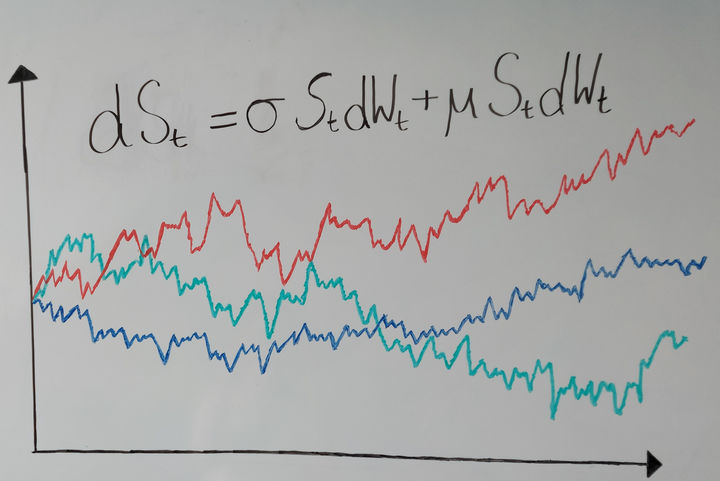# FSS 2024##### Advanced Topics in Mathematical Finance (MAC 557)

The course “Advanced Topics in Mathematical Finance” presents the foundational theory of mathematical finance in continuous time, including no arbitrage theory and pricing/hedging of financial derivatives, as well as a selection of more advanced topics, like volatility modeling and portfolio optimization.##### Stochastic Calculus (MAA 519)

The course “Stochastic Calculus” develops the mathematical theory required for probabilistic modeling of real-world phenomena, as it is used in various areas like mathematical finance, engineering and social science. In particular, we study stochastic integration with respect to Brownian motion, stochastic differential equations, change of measures, and martingale representation theorems.